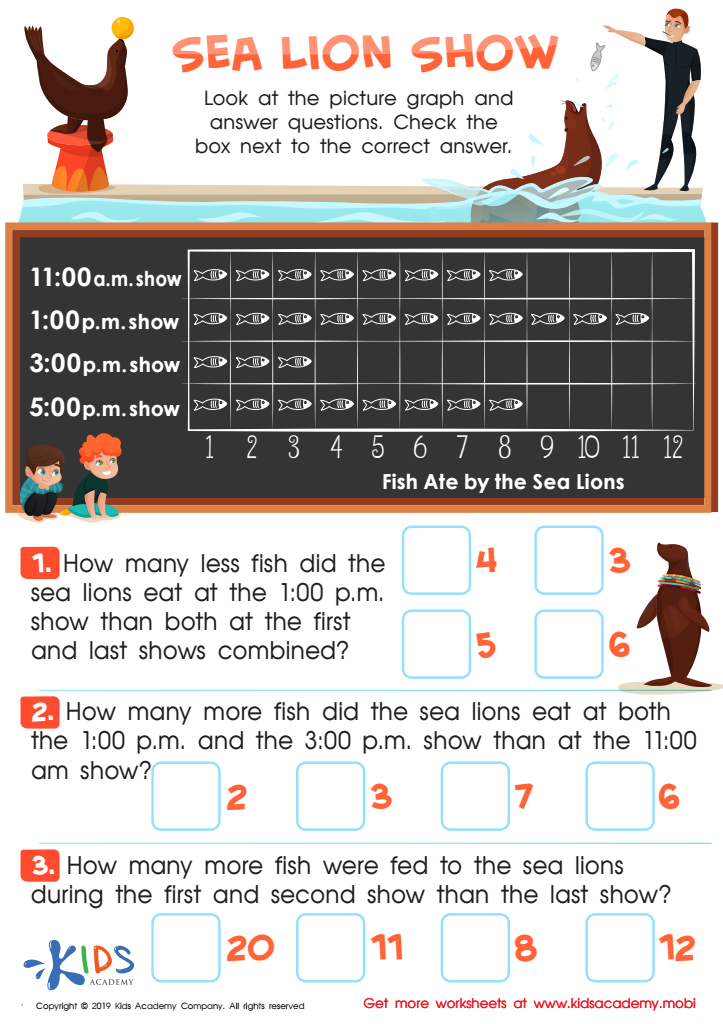# Extra Challenge Online Graphs Worksheets for Grade 3

10 filtered results

Clear all filters
Favorites
Online only

10 filtered results

Difficulty Level

Standards
Favorites
Online only
• Online only
• Graphs
• Extra Challenge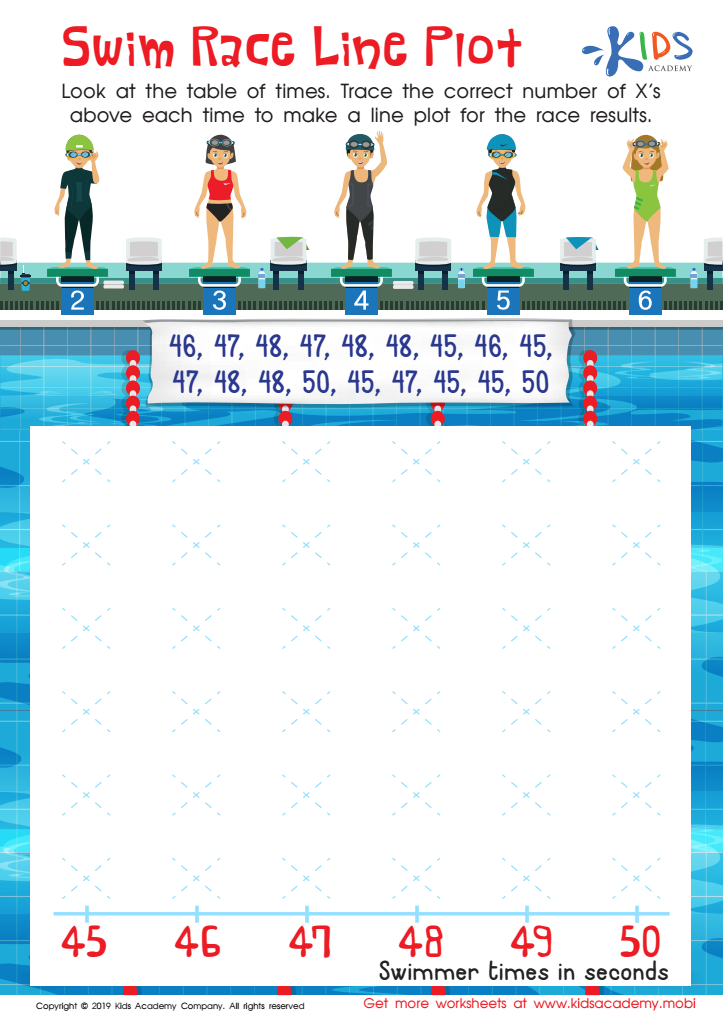Swim Race Line Plot Worksheet
Kindergartners can benefit from extra help with math, particularly line plots. This worksheet can make the concept easier to understand. Together, study the table of times; then, guide the kids in tracing the correct number of x's above each time to make a line plot for the race result.
Swim Race Line Plot Worksheet
Worksheet
Swim Race Line Plot Worksheet
Children are great learners, and they soak up everything you teach them in class. However, …
Graphs
Worksheet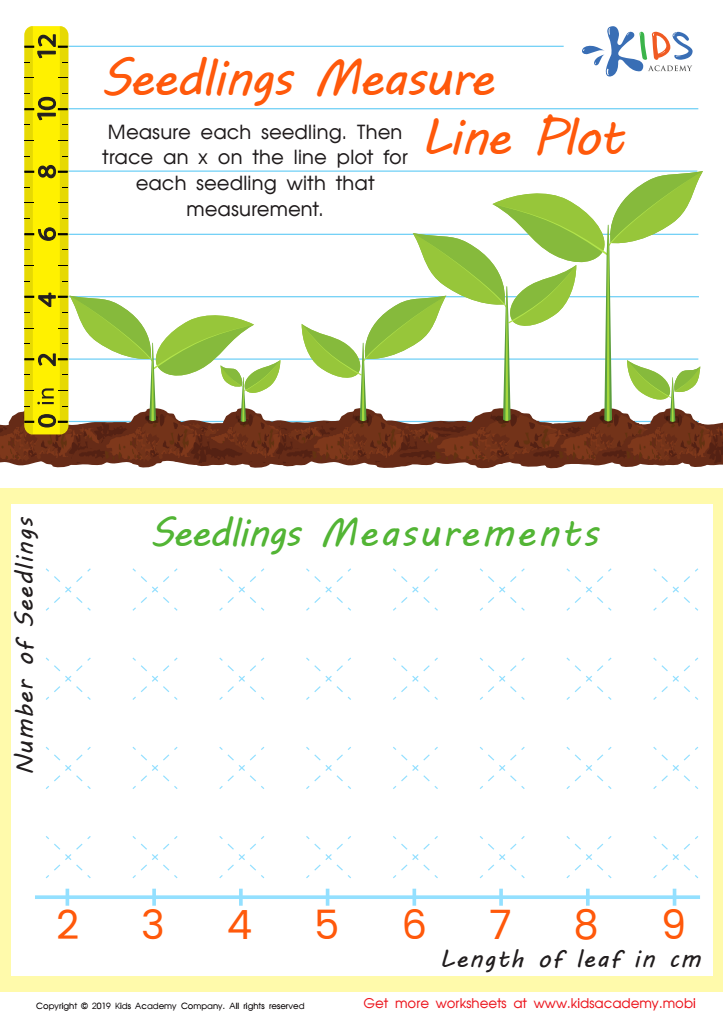Seedling Measure Line Plot Worksheet
Help your kids adjust to learning line plots with this fun tracing sheet. Measure each seedling in the picture, then trace an x on the line plot for each one. Assist them in completing this easy exercise!
Seedling Measure Line Plot Worksheet
Worksheet
Seedling Measure Line Plot Worksheet
If your kids are now learning line plots in school, this worksheet might be the …
Graphs
Worksheet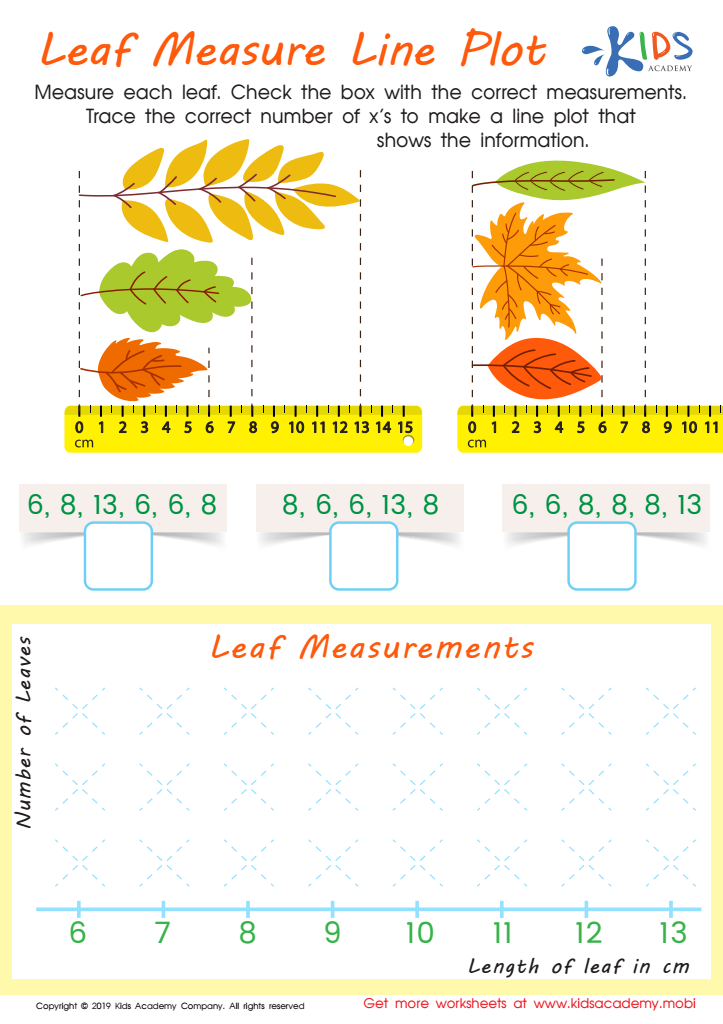Leaf Measure Line Plot Worksheet
Help your third grader measure each of the four leaves, and check the box with the correct measurements. Then, trace the right number of x's to make a line plot showing the data. This exercise will engage your students and make it easier for them to grasp the concept of line plots.
Leaf Measure Line Plot Worksheet
Worksheet
Leaf Measure Line Plot Worksheet
Line plots are not particularly difficult to understand, but your students might not be so …
Graphs
Worksheet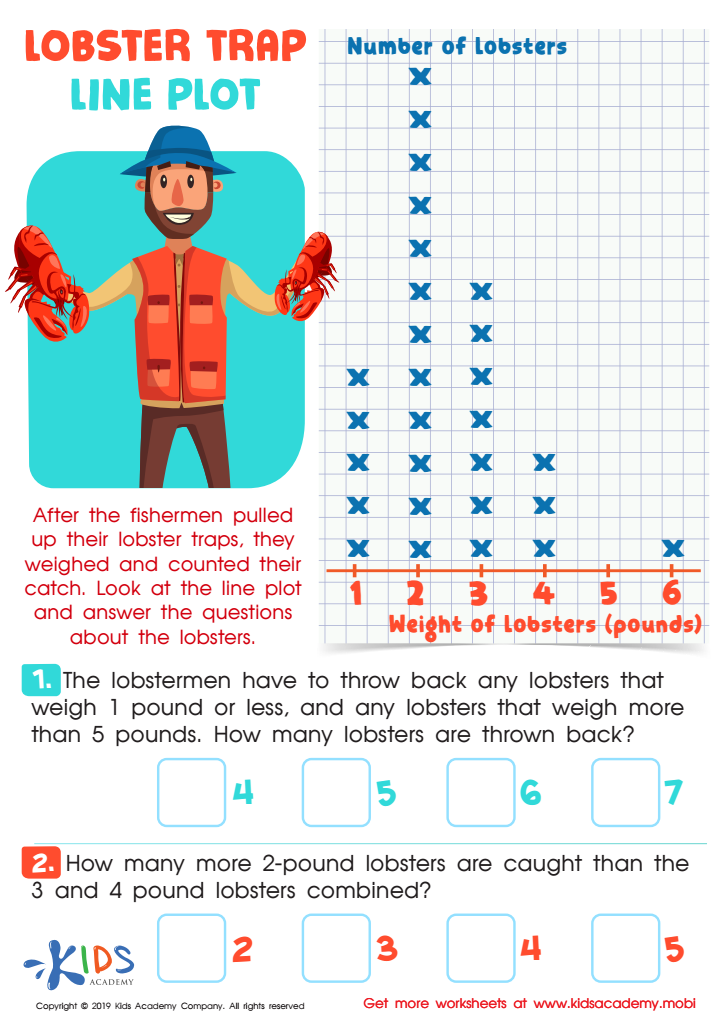Lobster Trap Line Plot Worksheet
If your kids are working on line plots, this worksheet is perfect for them! Show them the picture and read the instructions. Help them look at the line plot and answer the questions at the bottom. Learning math can be fun, so make it enjoyable together!
Lobster Trap Line Plot Worksheet
Worksheet
Lobster Trap Line Plot Worksheet
If your kids are now learning line plots in school, this worksheet might be the …